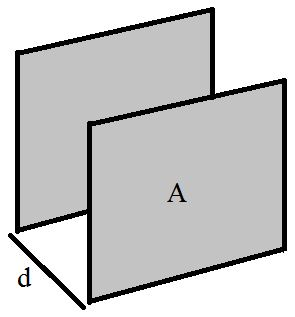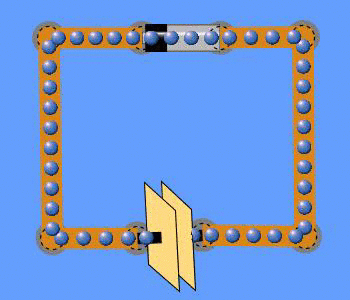# CapacitorFigure 1. Schematic of a capacitor, including two parallel plates with a surface area, A, and a separation distance, d. While not all capacitors are in this shape, they're often thought of as looking like this since it's the simplest geometry.Figure 2. An animation from a PhET simulation of a battery charging a capacitor until the current no longer flows through the circuit.

A capacitor is an electronic device that stores charge and energy. Capacitors can give off energy much faster than batteries can, resulting in much higher power density than batteries with the same amount of energy. Research into capacitors is ongoing to see if they can be used for storage of electrical energy for the electrical grid. While capacitors are old technology, supercapacitors are a new twist on this technology.

Capacitors are simply devices that consist of two conductors carrying equal but opposite charges. A simple parallel-plate capacitor consists of two equally-sized metal plates, known as electrodes, separated by an insulator, known as a dielectric, held parallel to each other. The capacitor is then integrated into an electric circuit. In a simple direct current circuit, each plate of the capacitor becomes oppositely charged over time due to the path of the electric current through the circuit. The battery drives charge in one direction such that one plate becomes positively charged and the other becomes negatively charged. This creates an electric field due to the buildup of equal and opposite charges, resulting in a potential difference, or voltage between the plates. Since the capacitance of the plates is constant, the voltage between the plates increases proportionally. As the charge on each plate increases, the voltage between the plates equals the voltage of the battery, at which point current will no longer flow through the circuit. This charging and discharging effect can be seen in Figure 2. Current can resume flowing if an alternate path is opened so that the capacitors can discharge itself or by using an alternating current so that the capacitor is periodically charged and discharged.

An important parameter for a capacitor is the capacitance, a measurement of the ability an object has to store charge. There are two main ways to calculate capacitance, using either the physical area of the plates or the voltage applied to the plates.

## Energy in a capacitor

The amount of energy stored by a capacitor is dependent on the capacitance and the voltage of the capacitor and is expressed as:

• is the voltage between the plates, measured in volts (V)
• is the capacitance of the capacitor, measured in farads (F)
• is the energy stored in the capacitor, measured in joules (J)

Increasing the capacitance or the voltage or both increases the amount of energy stored in the capacitor.

Alternatively, a dielectric can be added to the capacitor. A dielectric is an insulator placed between the electrodes. It increases the capacitance of the capacitor without needing to change its dimensions. This allows the capacitor to store more energy while still remaining small. How much of an increase is dependent to the material used for the dielectric.

## Uses

Capacitors do not have as high an energy density as batteries, meaning a capacitor cannot store as much energy as a comparable-sized battery. That said, the higher power capabilities of capacitors mean they are good for applications that require storing small amounts of energy, then releasing it very quickly. Le Mans Prototype race cars use capacitors to power electric motors in the front wheels. These capacitors are charged through regenerative braking, and allow all-wheel drive and extra power when exiting corners.

Capacitors are also used in many electronic devices that require a battery. This capacitor stores energy to prevent a loss of memory while the battery is being changed. A common (although not necessarily widely known) example is a camera flash charging. This is why two pictures can't be taken with a flash in rapid succession; the capacitor must build up the energy from the battery.

Moreover, capacitors play a key role in many practical circuits, primarily as current stabilizers and as components to aid in the conversion of alternating current to direct current in AC adapters. They are able to be used in this way due to the fact that capacitors are resistant to sudden changes in voltage, meaning that they have the ability to act as a buffer to store and withdraw electrical energy to maintain a stable output of current. Thus the capacitor is able to stabilize the fluctuating AC current by it's ability to hold and release electrical energy at different times.

Since capacitors store energy in electric fields, some researchers are working on developing supercapacitors in order to help with energy storage. This could prove useful in the transportation of energy or for storing and releasing energy from intermittent sources such as wind and solar power.

## Phet Simulation

The University of Colorado has graciously allowed us to use the following Phet simulation. Explore this simulation to see how gravitational potential energy and spring potential energy go back and forth and create a changing amount of kinetic energy (hint: click show energy before hanging a mass):

## Authors and Editors

Bethel Afework, Allison Campbell, Jordan Hanania, Braden Heffernan, James Jenden, Kailyn Stenhouse, Jason Donev
Last updated: June 4, 2018
Get Citation